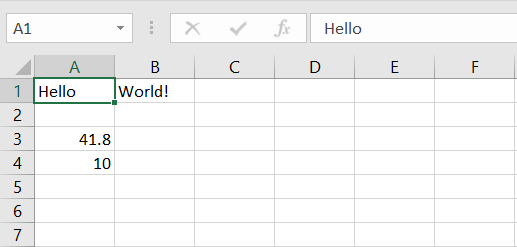# Reading and writing Excel files using the openpyxl module in Python

## Introduction

openpyxl is a Python library to read/write Excel 2010 xlsx/xlsm/xltx/xltm files.

It was born from lack of existing library to read/write natively from Python the Office Open XML format.

An excel file that we use for operation is called Workbook that contains a minimum of one Sheet and a maximum of tens of sheets.

One sheet consists of rows starting from 1 and columns starting from A.

Using the openpxyl library, we can perform various functions including adding sheets and data, manipulate and even delete said data.

Now that we know what we are dealing with, let us get started.

## Getting Started

The openpyxl does not come packaged with Python, which means we will have to download and install it using the PIP package manager. To do this, use launch your terminal and enter the command below.

pip install openpyxl

Once you have the library downloaded and installed, we’ll have to import it’s various modules to start working on the excel files.

Let us first import the workbook module from the openpyxl library

from openpyxl import Workbook

Now that we’ve made the necessary imports, we can work on creating and saving data to the excel sheet using the Workbook module.

## Creating and saving data in excel file

Firstly, we create an instance of the Workbook() class.

wb = Workbook()

Next, we create a sheet.

sheet = wb.active


It’s time to start adding data.

sheet['A1'] = "Hello"
sheet['A2'] = "World!"
sheet['A3'] = 41.80
sheet['A4'] = 10

Now that we’ve added some content, let us save and preview the file we’ve created.

wb.save("example.xlsx")

## OutputSimilarly, you can add values using cell coordinates instead of A1 or B1.

sheet.cell(row=2, column=2).value = 5

This will add 5 to B2.

## Reading data from an Excel file

Now that we’ve learnt how to write data into an excel file, let us now read data from an excel file.

This time we need not import the Workbook module, just importing openpyxl should do.

import openpyxl


In order to read from a file, we must first provide it’s location to the reader.

wb = openpyxl.load_workbook("example.xlsx")
sheet = wb.active

This will load up the excel file. We can now start reading data from it.

temp1 = sheet['A1']
temp2 = sheet['B1']
temp3 = sheet.cell(row = 3, column = 1)
temp4 = sheet.cell(row = 4, column = 1)
print(temp1.value, temp2.value, temp3.value, temp4.value)

## Output

Hello World! 41.8 10

## Example

from openpyxl import Workbook
import openpyxl

wb = Workbook()
sheet = wb.active
sheet['A1'] = "Hello"
sheet['B1'] = "World!"
sheet['A3'] = 41.80
sheet['A4'] = 10

wb.save("example.xlsx")

sheet = wb.active
temp1 = sheet['A1']
temp2 = sheet['B1']
temp3 = sheet.cell(row = 3, column = 1)
temp4 = sheet.cell(row = 4, column = 1)
print(temp1.value, temp2.value, temp3.value, temp4.value)

## Conclusion

You can now read and write excel files using Python!

There are a lot more features within the openpyxl library, you can add multiple data at once, build charts, display stats and much more!

If you are curious and want to learn more, check out their official documentation at https://pypi.org/project/openpyxl/.